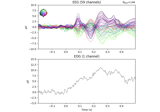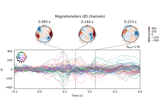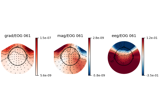# mne.preprocessing.EOGRegression#

Remove EOG artifact signals from other channels by regression.

Employs linear regression to remove signals captured by some channels, typically EOG, as described in . You can also choose to fit the regression coefficients on evoked blink/saccade data and then apply them to continuous data, as described in .

Parameters:
picks

Channels to include. Slices and lists of integers will be interpreted as channel indices. In lists, channel type strings (e.g., ```['meg', 'eeg']```) will pick channels of those types, channel name strings (e.g., `['MEG0111', 'MEG2623']` will pick the given channels. Can also be the string values “all” to pick all channels, or “data” to pick data channels. None (default) will pick good data channels. Note that channels in `info['bads']` will be included if their names or indices are explicitly provided.

exclude`list` | ‘bads’

List of channels to exclude from the regression, only used when picking based on types (e.g., exclude=”bads” when picks=”meg”). Specify `'bads'` (the default) to exclude all channels marked as bad.

picks_artifact

Channel picks to use as predictor/explanatory variables capturing the artifact of interest (default is “eog”).

proj`bool`

Whether to automatically apply SSP projection vectors before fitting and applying the regression. Default is `True`.

Notes

New in version 1.2.

References

Attributes:
coef_`ndarray`, shape (n, n)

The regression coefficients. Only available after fitting.

info_`Info`

Channel information corresponding to the regression weights. Only available after fitting.

picks

Channels to perform the regression on.

exclude`list` | ‘bads’

Channels to exclude from the regression.

picks_artifact

The channels designated as containing the artifacts of interest.

proj`bool`

Whether projections will be applied before performing the regression.

Methods

 `apply`(inst[, copy]) Apply the regression coefficients to data. `fit`(inst) Fit EOG regression coefficients. `plot`([ch_type, sensors, show_names, mask, ...]) Plot the regression weights of a fitted EOGRegression model. `save`(fname[, overwrite]) Save the regression model to an HDF5 file.
apply(inst, copy=True)[source]#

Apply the regression coefficients to data.

Parameters:
inst

The data on which to apply the regression.

copy`bool`

If `True`, data will be copied. Otherwise data may be modified in place. Defaults to `True`.

Returns:
inst

A version of the data with the artifact channels regressed out.

Notes

Only works after `.fit()` has been used.

References

Examples using `apply`:Repairing artifacts with regression

Repairing artifacts with regressionPreprocessing optically pumped magnetometer (OPM) MEG data

Preprocessing optically pumped magnetometer (OPM) MEG dataReduce EOG artifacts through regression

Reduce EOG artifacts through regression
fit(inst)[source]#

Fit EOG regression coefficients.

Parameters:
inst

The data on which the EOG regression weights should be fitted.

Returns:
self`EOGRegression`

The fitted `EOGRegression` object. The regression coefficients are available as the `.coef_` and `.intercept_` attributes.

Notes

If your data contains EEG channels, make sure to apply the desired reference (see `mne.set_eeg_reference()`) before performing EOG regression.

Examples using `fit`:Preprocessing optically pumped magnetometer (OPM) MEG data

Preprocessing optically pumped magnetometer (OPM) MEG data
plot(ch_type=None, sensors=True, show_names=False, mask=None, mask_params=None, contours=6, outlines='head', sphere=None, image_interp='cubic', extrapolate='auto', border='mean', res=64, size=1, cmap=None, vlim=(None, None), cnorm=None, axes=None, colorbar=True, cbar_fmt='%1.1e', title=None, show=True)[source]#

Plot the regression weights of a fitted EOGRegression model.

Parameters:
ch_type‘mag’ | ‘grad’ | ‘planar1’ | ‘planar2’ | ‘eeg’ | `None`

The channel type to plot. For `'grad'`, the gradiometers are collected in pairs and the RMS for each pair is plotted. If `None` the first available channel type from order shown above is used. Defaults to `None`.

sensors

Whether to add markers for sensor locations. If `str`, should be a valid matplotlib format string (e.g., `'r+'` for red plusses, see the Notes section of `plot()`). If `True` (the default), black circles will be used.

show_names

If `True`, show channel names next to each sensor marker. If callable, channel names will be formatted using the callable; e.g., to delete the prefix ‘MEG ‘ from all channel names, pass the function `lambda x: x.replace('MEG ', '')`. If `mask` is not `None`, only non-masked sensor names will be shown.

mask`ndarray` of `bool`, shape (n_channels,) | `None`

Array indicating channel(s) to highlight with a distinct plotting style. Array elements set to `True` will be plotted with the parameters given in `mask_params`. Defaults to `None`, equivalent to an array of all `False` elements.

Additional plotting parameters for plotting significant sensors. Default (None) equals:

```dict(marker='o', markerfacecolor='w', markeredgecolor='k',
linewidth=0, markersize=4)
```
contours

The number of contour lines to draw. If `0`, no contours will be drawn. If a positive integer, that number of contour levels are chosen using the matplotlib tick locator (may sometimes be inaccurate, use array for accuracy). If array-like, the array values are used as the contour levels. The values should be in µV for EEG, fT for magnetometers and fT/m for gradiometers. If `colorbar=True`, the colorbar will have ticks corresponding to the contour levels. Default is `6`.

outlines‘head’ | `dict` | `None`

The outlines to be drawn. If ‘head’, the default head scheme will be drawn. If dict, each key refers to a tuple of x and y positions, the values in ‘mask_pos’ will serve as image mask. Alternatively, a matplotlib patch object can be passed for advanced masking options, either directly or as a function that returns patches (required for multi-axis plots). If None, nothing will be drawn. Defaults to ‘head’.

sphere`float` | array_like | instance of `ConductorModel` | `None` | ‘auto’ | ‘eeglab’

The sphere parameters to use for the head outline. Can be array-like of shape (4,) to give the X/Y/Z origin and radius in meters, or a single float to give just the radius (origin assumed 0, 0, 0). Can also be an instance of a spherical `ConductorModel` to use the origin and radius from that object. If `'auto'` the sphere is fit to digitization points. If `'eeglab'` the head circle is defined by EEG electrodes `'Fpz'`, `'Oz'`, `'T7'`, and `'T8'` (if `'Fpz'` is not present, it will be approximated from the coordinates of `'Oz'`). `None` (the default) is equivalent to `'auto'` when enough extra digitization points are available, and (0, 0, 0, 0.095) otherwise.

New in version 0.20.

Changed in version 1.1: Added `'eeglab'` option.

image_interp`str`

The image interpolation to be used. Options are `'cubic'` (default) to use `scipy.interpolate.CloughTocher2DInterpolator`, `'nearest'` to use `scipy.spatial.Voronoi` or `'linear'` to use `scipy.interpolate.LinearNDInterpolator`.

extrapolate`str`

Options:

• `'box'`

Extrapolate to four points placed to form a square encompassing all data points, where each side of the square is three times the range of the data in the respective dimension.

• `'local'` (default for MEG sensors)

Extrapolate only to nearby points (approximately to points closer than median inter-electrode distance). This will also set the mask to be polygonal based on the convex hull of the sensors.

• `'head'` (default for non-MEG sensors)

Extrapolate out to the edges of the clipping circle. This will be on the head circle when the sensors are contained within the head circle, but it can extend beyond the head when sensors are plotted outside the head circle.

Changed in version 0.21:

• The default was changed to `'local'` for MEG sensors.

• `'local'` was changed to use a convex hull mask

• `'head'` was changed to extrapolate out to the clipping circle.

border`float` | ‘mean’

Value to extrapolate to on the topomap borders. If `'mean'` (default), then each extrapolated point has the average value of its neighbours.

New in version 0.20.

res`int`

The resolution of the topomap image (number of pixels along each side).

size`float`

Side length of each subplot in inches.

cmapmatplotlib colormap | (colormap, `bool`) | ‘interactive’ | `None`

Colormap to use. If `tuple`, the first value indicates the colormap to use and the second value is a boolean defining interactivity. In interactive mode the colors are adjustable by clicking and dragging the colorbar with left and right mouse button. Left mouse button moves the scale up and down and right mouse button adjusts the range. Hitting space bar resets the range. Up and down arrows can be used to change the colormap. If `None`, `'Reds'` is used for data that is either all-positive or all-negative, and `'RdBu_r'` is used otherwise. `'interactive'` is equivalent to `(None, True)`. Defaults to `None`.

Warning

Interactive mode works smoothly only for a small amount of topomaps. Interactive mode is disabled by default for more than 2 topomaps.

vlim`tuple` of length 2

Colormap limits to use. If a `tuple` of floats, specifies the lower and upper bounds of the colormap (in that order); providing `None` for either entry will set the corresponding boundary at the min/max of the data. Defaults to `(None, None)`.

cnorm

How to normalize the colormap. If `None`, standard linear normalization is performed. If not `None`, `vmin` and `vmax` will be ignored. See Matplotlib docs for more details on colormap normalization, and the ERDs example for an example of its use.

axesinstance of `Axes` | `list` of `Axes` | `None`

The axes to plot to. If `None`, a new `Figure` will be created with the correct number of axes. If `Axes` are provided (either as a single instance or a `list` of axes), the number of axes provided must match the number of `times` provided (unless `times` is `None`).Default is `None`.

colorbar`bool`

Plot a colorbar in the rightmost column of the figure.

cbar_fmt`str`

Formatting string for colorbar tick labels. See Format Specification Mini-Language for details.

title

The title of the generated figure. If `None` (default), no title is displayed.

show`bool`

Show the figure if `True`.

Returns:
figinstance of `matplotlib.figure.Figure`

Figure with a topomap subplot for each channel type.

Notes

New in version 1.2.

Examples using `plot`:Repairing artifacts with regression

Repairing artifacts with regressionReduce EOG artifacts through regression

Reduce EOG artifacts through regression
save(fname, overwrite=False)[source]#

Save the regression model to an HDF5 file.

Parameters:
fname`str`

The file to write the regression weights to. Should end in `.h5`.

overwrite`bool`

If True (default False), overwrite the destination file if it exists.

## Examples using `mne.preprocessing.EOGRegression`#Repairing artifacts with regression

Repairing artifacts with regressionPreprocessing optically pumped magnetometer (OPM) MEG data

Preprocessing optically pumped magnetometer (OPM) MEG dataReduce EOG artifacts through regression

Reduce EOG artifacts through regression